﻿ Creating Outlines and Trees with a WinForms Data Grid Control | ComponentOne# Creating Outlines and Trees with a WinForms Data Grid Control

November 25, 2015 Update: See our newer version of this post here: Creating Outlines and Trees with the C1FlexGrid Control, Updated

One of the unique and popular features of the FlexGrid for WinForms control is the ability to add hierarchical grouping to regular unstructured data.

To achieve this, the C1FlexGrid introduces the concept of Node rows. Node rows do not contain regular data. Instead, they act as headers under which similar data is grouped, exactly like nodes in a regular TreeView control. Like nodes in a TreeView control, node rows can be collapsed and expanded, hiding or showing the data they contain. Also like nodes in a TreeView control, node rows have a Level property that defines the node hierarchy. Lower level nodes contain higher level nodes.

For example, suppose you had a grid showing customer name, country, city, and sales amounts. This typical grid would normally look like this: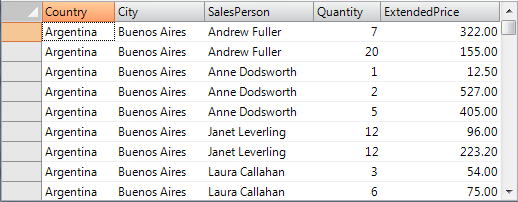All the information is there, but it's hard to see the total sales for each country or customer. You could use the C1FlexGrid's outlining features to group the data by country (level 0), then by city within each country (level 1), then by customer within each city (level 2). Here is the same grid with after adding the outline: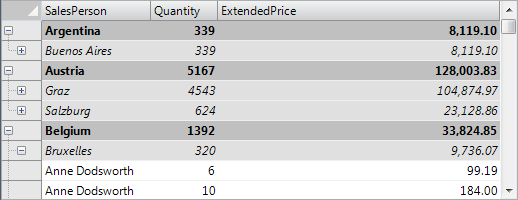This grid shows the same information as the previous one (it is bound to the same data source), but it adds a tree where each node contains a summary of the data below it. Nodes can be collapsed to show only the summary, or expanded to show the detail. Note that each node row can show summaries for more than one column (in this case, total units sold and total amount).

In this article, we will walk you through the process of turning a regular grid into a richer outline grid.

Loading data into an outline grid is exactly the same as loading it into a regular grid. If your data source is available at design time, you can use the Visual Studio Property Window to set the grid's DataSource property and bind the grid to the data without writing any code.

If the data source is not available at design time, you can set the grid's DataSource property in code. The data binding code typically looks like this:

``````public Form1()
{
InitializeComponent();

// get data
var fields = @"
Country,
City,
SalesPerson,
Quantity,
ExtendedPrice";
var sql = string.Format("SELECT {0} FROM Invoices ORDER BY {0}", fields);
var da = new OleDbDataAdapter(sql, GetConnectionString());
da.Fill(_dt);

**// bind grid to data
this.\_flex.DataSource = \_dt;**

// format ExtendedPrice column
_flex.Cols["ExtendedPrice"].Format = "n2";
}
``````

The code uses an OleDbDataAdapter to fill a DataTable with data, then assigns the DataTable to the grid's DataSource property.

After running this code, you would get the "regular grid" shown in the first image. To turn this regular grid into the outline grid shown in the second image, we need to insert the node rows that make up the outline.

### Creating Node Rows

Node rows are almost identical to regular rows, except for the following:

• Node rows are not data bound. When the grid is bound to a data source, each regular row corresponds to an item in the data source. Node rows do not. Instead, they exist to group regular rows that contain similar data.
• Node rows can be collapsed or expanded. When a node row is collapsed, all its data and child nodes are hidden. If the outline tree is visible, users can collapse and expand nodes using the mouse or the keyboard. If the outline tree is not visible, then nodes can only be expanded or collapsed using code.

To determine whether a row is a node or not, you can use the IsNode property:

``````var row = _flex.Rows[rowIndex];
if (row.IsNode)
{
// row is a node
var node = row.Node;
DoSomethingWithTheNode(node);
}
else
{
// this row is not a node
}
``````

Node rows can be created in three ways:

1. Use the Rows.InsertNode method. This will insert a new node row at a specified index. Once the node row has been created, you can use it like you would any other row (set the data for each column, apply styles, etc). This is the 'low-level' way of inserting totals and building outlines. It gives the most control and flexibility and is demonstrated below.
2. Use the Subtotal method. This method scans the entire grid and automatically inserts node rows with optional subtotals at locations where the grid data changes. This is the 'high-level' way of inserting totals and building outlines. It requires very little code, but makes some assumptions about how the data is structured on the grid and what the outline should look like.
3. If the grid is unbound, then you can turn regular rows into node rows by setting the IsNode property to true. Note that this only works when the grid is unbound. Trying to turn a regular data bound row into a node will cause the grid to throw an exception.

The code below shows how you could implement a GroupBy method that inserts node rows grouping identical values on a given column.

``````// group on a given column inserting nodes of a given level
void GroupBy(string columnName, int level)
{
object current = null;
for (int r = \_flex.Rows.Fixed; r < \_flex.Rows.Count; r  )
{
if (!_flex.Rows[r].IsNode)
{
var value = _flex[r, columnName];
if (!object.Equals(value, current))
{
// value changed: insert node
**_flex.Rows.InsertNode(r, level);**

// show group name in first scrollable column
\_flex[r, \_flex.Cols.Fixed] = value;

// update current value
current = value;
}
}
}
}

``````

The code scans all the columns, skipping existing node rows (so it can be called to add several levels of nodes), and keeps track of the current value for the column being grouped on. When the current value changes, a node row is inserted showing the new in the first scrollable column.

Back to our example, you could use this method to create a two-level outline by calling:

``````void \_btnGroupCountryCity\_Click(object sender, EventArgs e)
{
GroupBy("Country", 0);
GroupBy("City", 1);
}
``````

Very simple, but there are some caveats. First, the method assumes that the data is sorted according to the outline structure. In this example, if the data were sorted by SalesPerson instead of by Country, the outline would have several level-0 nodes for each country, which probably is not what you want.

Also, the GroupBy method may insert may rows, which would cause the grid to flicker. To avoid this, you would normally set the Redraw property to false before making the updates and set it back to true when done.

To handle these issues, the code that creates the outline should be re-written as follows:

``````void \_btnGroupCountryCity\_Click(object sender, EventArgs e)
{
// suspend redrawing while updating
using (new DeferRefresh(_flex))
{
// restore original sort (by Country, City, etc.)
ResetBinding();

// group by Country, City
GroupBy("Country", 0);
GroupBy("City", 1);
}
}
``````

The DeferRefresh class is a simple utility that sets the grid's Redraw property to false and restores its original value when it is disposed. This ensures that Redraw is properly restored even when exceptions happen during the updates. Here is the implementation of the DeferRefresh class:

``````/// Utility class used to encapsulate grid lengthy operations in a Redraw block.
/// This avoids flicker and ensures the Redraw property is reset properly in case
/// an exception is thrown during the operation.
class DeferRefresh : IDisposable
{
C1FlexGrid _grid;
bool _redraw;
public DeferRefresh(C1FlexGrid grid)
{
_grid = grid;
_redraw = grid.Redraw;
**grid.Redraw = false;**
}
public void Dispose()
{
**\_grid.Redraw = \_redraw;**
}
}
``````

The BindGrid method ensures that the grid is sorted in the order required by our outline structure. In our example, the sort order is by Country, City, and SalesPerson. The code looks like this:

``````// unbind and re-bind grid in order to reset everything
void ResetBinding()
{
// unbind grid
_flex.DataSource = null;

// reset any custom sorting
_dt.DefaultView.Sort = string.Empty;

// re-bind grid
\_flex.DataSource = \_dt;

// format ExtendedPrice column
_flex.Cols["ExtendedPrice"].Format = "n2";

// auto-size the columns to fit their content
_flex.AutoSizeCols();
}
``````

If you run this code now, you will notice that the node rows are created as expected, but the outline tree is not visible, so you can't expand and collapse the nodes. The outline tree is described in the next section.

## Outline Tree

The outline tree is very similar to the one you see in a regular TreeView control. It shows an indented structure with collapse/expand icons next to each node row so the user can expand and collapse the outline to see the desired level of detail.

The outline tree can be displayed in any column, defined by the Tree.Column property. By default, this property is set to -1, which causes the tree not to be displayed at all. To show the outline tree in the example given above, you would use this code:

``````void \_btnTreeCountryCity\_Click(object sender, EventArgs e)
{
using (new DeferRefresh(_flex))
{
// group by country and city as before
\_btnGroupCountryCity\_Click(this, EventArgs.Empty);

**// show outline tree
_flex.Tree.Column = 0;
**
// autosize to accommodate tree
\_flex.AutoSizeCol(\_flex.Tree.Column);

// collapse detail nodes
_flex.Tree.Show(1);
}
}
``````

The code calls the previous method to build the outline, then sets the Tree.Column property to zero in order to show the outline tree in the first column. It also calls the AutoSizeCol method to ensure that the column is wide enough to accommodate the outline tree. Finally, it calls the Tree.Show method to display all level-0 nodes (cities in this case) and hide all the detail.

The Tree property returns a reference to a GridTree object that exposes several methods and properties used to customize the outline tree. The main ones are listed below:

• Column: Gets or sets the index of the column that contains the outline tree. Setting this property to -1 causes the outline tree to be hidden from the users.
• Indent: Gets or sets the indent, in pixels, between adjacent node levels. Higher indent levels cause the tree to become wider.
• Style: Gets or sets the type of outline tree to display. Use this property to determine whether the tree should include a button bar at the top to allow users to collapse/expand the entire tree, whether lines and/or symbols should be displayed, and whether lines should be displayed connecting the tree to data rows as well as node rows.
• LineColor: Gets or sets the color of the tree's connecting lines.
• LineStyle: Gets or sets the style of the tree's connecting lines.

For example, by changing the code above to include these two lines:

``````// show outline tree
_flex.Tree.Column = 0;
_flex.Tree.Style = TreeStyleFlags.CompleteLeaf;
_flex.Tree.LineColor = Color.White;
_flex.Tree.Indent = 30;
``````

The outline tree would change as follows: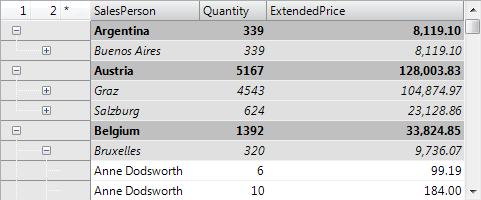Notice the buttons labeled "1", "2", and "*" on the top left cell. Clicking these buttons would cause the entire tree to collapse or expand to the corresponding level. Also notice the much wider indentation and the lines connecting the tree to regular rows ("Anne Dodsworth") as well as to node rows.

So far we have covered the creation of node rows and outline trees. To make the outlines really useful, however, the node rows should include summary information for the data they contain.

If you create an outline tree using the Subtotal method, then the subtotals are added automatically. This will be described in a later section.

If you created the outline tree using the Rows.InsertNode method as described above, then you should use the Aggregate method to calculate the subtotals for each group of rows and insert the result directly into the node rows.

The Subtotal method listed below shows how to do this:

``````// add subtotals to each node at a given level
{
// get column we are going to total on
int colIndex = _flex.Cols.IndexOf(colName);

// scan rows looking for nodes at the right level
for (int r = \_flex.Rows.Fixed; r < \_flex.Rows.Count; r  )
{
if (_flex.Rows[r].IsNode)
{
var node = _flex.Rows[r].Node;
if (node.Level == level)
{
// found a node, calculate the sum of extended price
var range = node.GetCellRange();
**var sum = _flex.Aggregate(AggregateEnum.Sum,
range.r1, colIndex, range.r2, colIndex,
AggregateFlags.ExcludeNodes);**

// show the sum on the grid
// (will use the column format automatically)
_flex[r, colIndex] = sum;
}
}
}
}
``````

The AddSubtotals method scans the grid rows looking for node rows. When a node row of the desired level is found, the method uses the Node.GetCellRange method to retrieve the node's child rows. Then it uses the Aggregate method to calculate the sum of the values on the target column over the entire range. The call to Aggregate includes the ExcludeNodes flag to avoid double-counting existing nodes. Once the subtotal has been calculated, it is assigned to the node row's cell with the usual _flex[row, col] indexer.

Note that this does not affect the data source in any way, since node rows are not bound to the data.

Note also that the method can be used to add multiple totals to each node row. In this example, we will add totals for the Quantity and ExtendedPrice columns. In addition to sums, you could add other aggregates such as average, maximum, minimum, etc.

We can now use this method to create a complete outline, with node rows, outline tree, and subtotals:

``````void \_btnTreeCountryCity\_Click(object sender, EventArgs e)
{
using (new DeferRefresh(_flex))
{
// restore original sort (by Country, City, SalesPerson)
ResetBinding();

// group by Country, City
GroupBy("Country", 0); // group by country (level 0)
GroupBy("City", 1);    // group by city (level 1)

// add totals per Country, City
AddSubtotals(0, "ExtendedPrice");  // extended price per country (level 0)
AddSubtotals(0, "Quantity");       // quantity per country (level 0)
AddSubtotals(1, "ExtendedPrice");  // extended price per city (level 1)
AddSubtotals(1, "Quantity");       // quantity per city (level 1)

// show outline tree
_flex.Tree.Column = 0;
\_flex.AutoSizeCol(\_flex.Tree.Column);
_flex.Tree.Show(1);
}
}

``````

If you run the project now, you will see a tree with node rows that show the total quantity and amount sold for each country and city. This is very nice, but there is a little problem. If you expand any of the node rows, you will see a lot of duplicate values. All rows under a given city node have the same country and city: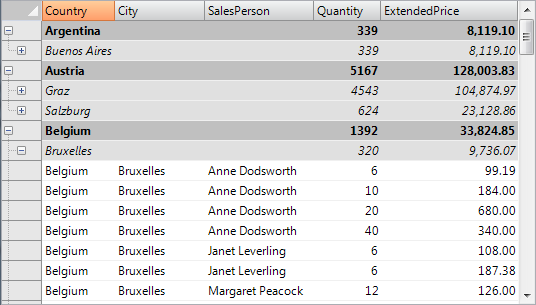This is correct, but it is also a waste of screen real estate. Eliminating these duplicate values is easy, all you have to do is set the Width of the columns that are being grouped on to zero. When you do that, however, you should remember to set the grid's AllowMerging property to Nodes, so the text assigned to the node rows will spill into the visible columns. (Another option would be to assign the node text to the first visible column, but merging is usually a better solution because it allows you to use longer text for the node rows).

Here is the revised code and the final result:

``````void \_btnTreeCountryCity\_Click(object sender, EventArgs e)
{
using (new DeferRefresh(_flex))
{
// restore original sort (by Country, City, SalesPerson)
ResetBinding();

// group by Country, City
GroupBy("Country", 0); // group by country (level 0)
GroupBy("City", 1);    // group by city (level 1)

// hide columns that we grouped on
// (they only have duplicate values which already appear on the tree nodes)
// (but don't make them invisible, that would also hide the node text)
_flex.Cols["Country"].Width = 0;
_flex.Cols["City"].Width = 0;

// allow node content to spill onto next cell
_flex.AllowMerging = AllowMergingEnum.Nodes;

// add totals per Country, City
AddTotals(0, "ExtendedPrice");  // extended price per country (level 0)
AddTotals(0, "Quantity");       // quantity per country (level 0)
AddTotals(1, "ExtendedPrice");  // extended price per city (level 1)
AddTotals(1, "Quantity");       // quantity per city (level 1)

// show outline tree
_flex.Tree.Column = 0;
\_flex.AutoSizeCol(\_flex.Tree.Column);
_flex.Tree.Show(1);
}
}

``````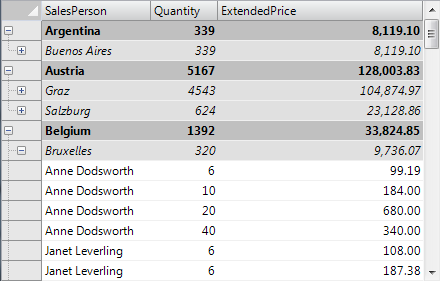The Country and City columns are now invisible, but their values still appear in the node rows. Collapsing the tree shows totals for each country and city.

## Using the Subtotal Method

We mentioned earlier that you could also create trees using the C1FlexGrid's Subtotal method. The Subtotal method performs the same tasks as the GroupBy and AddSubtotals methods described above, except it does both things in a single step and is therefore a little more efficient.

The code below shows how you can use the Subtotal method to accomplish the same thing we did before, only a little faster and without using any helper methods:

``````void \_btnTreeCountryCity\_Click(object sender, EventArgs e)
{
using (new DeferRefresh(_flex))
{
// restore original sort (by Country, City, SalesPerson)
ResetBinding();

// group and total by country and city
_flex.Subtotal(AggregateEnum.Sum, 0, "Country", "ExtendedPrice");
_flex.Subtotal(AggregateEnum.Sum, 0, "Country", "Quantity");
_flex.Subtotal(AggregateEnum.Sum, 1, "City", "ExtendedPrice");
_flex.Subtotal(AggregateEnum.Sum, 1, "City", "Quantity");

// hide columns that we grouped on
// (they only have duplicate values which already appear on the tree nodes)
// (but don't make them invisible, that would also hide the node text)
_flex.Cols["Country"].Width = 0;
_flex.Cols["City"].Width = 0;
_flex.AllowMerging = AllowMergingEnum.Nodes;

// show outline tree
_flex.Tree.Column = 0;
\_flex.AutoSizeCol(\_flex.Tree.Column);
_flex.Tree.Show(1);
}
}
``````

The Subtotal method is very convenient and flexible. It has a number of overloads that allow you to specify which columns should be grouped on and totaled on by index or by name, whether to include a caption in the node rows that it inserts, how to perform the grouping, and so on. The summary below describes the overloads available:

1. Subtotal(AggregateEnum aggType)
This version of the method takes only one aggregate type as a parameter. It is useful only for removing existing subtotals before inserting new ones. In this case, the aggType parameter is set to AggregateEnum.Clear.
2. Subtotal(AggregateEnum aggType, int groupBy, int totalOn)
Subtotal(
AggregateEnum aggType, string groupBy, string totalOn) These are the most commonly used overloads. The parameters are the type of aggregate to insert and the columns to group by and total on. The columns may be referenced by index or by name. The latter is the one we used in the example above.
3. Subtotal(AggregateEnum aggType, int groupBy, int totalOn, string caption)
Subtotal(
AggregateEnum aggType, string groupBy, string totalOn, string caption) These overloads add an extra caption parameter. The caption parameter determines the text that is added to the new node rows to identify the value being grouped on. By default, the value being grouped on is shown, so if you are grouping by country, the node rows would show "Argentina", "Brazil", and so on. If you set the caption parameter to a string such as "Country: {0}", then the node rows would show "Country: Argentina" instead.
4. Subtotal(AggregateEnum aggType, int groupFrom, int groupTo, int totalOn, string caption)
Subtotal(
AggregateEnum aggType, string groupFrom, string groupTo, string totalOn, string caption) These overloads separate the groupBy parameter into two: groupFrom and groupTo. By default, the Subtotal method inserts a node row whenever the value of the groupBy or any previous column changes.
For example, if you a row has the same value in the "City" column as the previous row, but a different value in the "Country" column, then the Subtotal method assumes the rows should be in different groups and inserts a new node row even though the value of the groupBy column is the same. These aggregates let you override that behavior and specify the range of columns that should be considered when identifying a group.

## Outline Maintenance

So far we have discussed how to create outlines with trees and totals using the high-level Subtotal method as well as lower-level Rows.InsertNode and Aggregate methods.

At this point, it is important to remember that the outline tree is created based on the data, but is not bound to it in any way, and is not automatically maintained when there are changes to the grid or to the data.

If the user modifies a value in the "ExtendedPrice" column for example, the subtotals will not be automatically updated. If the user sorts the grid, the data will be refreshed and the subtotals will disappear.

There are two common ways to maintain the outlines:

1. Prevent the user from making any changes that would invalidate the outline. This is the easiest option. You would set the grid's AllowEditing, AllowDragging, and AllowSorting properties to false and prevent any changes that would affect the outline.
2. Update the outline when there are changes to the data or to the grid. You would attach handlers to the grid's AfterDataRefresh, AfterSort, and AfterEdit events and re-generate the outline appropriately.

Option 2 is usually more interesting since it provides a quick and simple tool for dynamic data analysis. This approach is illustrated by the Analyze sample provided with the C1FlexGrid. The sample creates an initial outline and allows users to reorder the columns. When the column order changes, the sample automatically re-sorts the data and re-creates the outline. The user can easily create simple reports showing sales by country, by product, by salesperson, and so on.

## Using the Node class

The Node class provides a number of methods and properties that can be used to create and manage outline trees. Many of these methods and properties are based on the standard TreeView object model, so they should be familiar to most developers.

To obtain a Node object, you can either:

Use the return value of the Rows.InsertNode method:

``````var node = _flex.Rows.InsertNode(index, level);
``````

Or you can retrieve the node for an existing row using the row's Node property:

``````var node = _flex.Rows[index].IsNode
? _flex.Rows[index].Node
: null;
``````

Either way, once you have a Node object you can manipulate it using the following properties and methods:

• Level: Gets or sets the node level in the outline tree.
• Data: Gets or sets the value in the cell defined by Node.Row and the Tree.Column.
• Image: Gets or sets the image in the cell defined by Node.Row and the Tree.Column.
• Checked: Gets or sets the check state of the cell defined by Node.Row and the Tree.Column.
• Collapsed/Expanded: Gets or sets the node's collapsed/expanded state.

You can also explore the outline structure using the following methods:

• GetCellRange(): Gets a CellRange object that described the range of rows that belong to this node.
• Children: Gets the number of child nodes under this node.
• Nodes: Gets a node array containing this node's child nodes.
• GetNode: Gets the node that has a given relationship to this node (parent, first child, next sibling, and so on).

The discussion above focused on bound scenarios, where the grid is attached to a data source that provides the data. You can also create trees and outlines in unbound scenarios. Things are actually somewhat simpler in this case, since you can turn any row into a node row by setting its IsNode property to true.

If the grid is unbound, it owns all the data that is displayed, and you do things that are not possible when a data source owns the data. For example, you can move nodes around the tree using the Node.Move method as shown by the TreeNode sample provided with the C1FlexGrid.

Using nodes in an unbound grid is very similar to using nodes in a regular TreeView control.